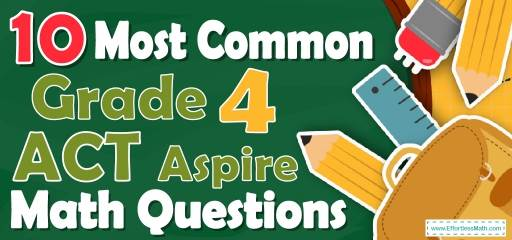# 10 Most Common 4th Grade ACT Aspire Math QuestionsWant to help your 4th-grade student prepare for the ACT Aspire Math test? Want a preview of the most common mathematics questions on the 4th Grade ACT Aspire Math test? If so, then you are in the right place.

The mathematics section of 4th Grade ACT Aspire can be a challenging area for many test-takers, but with enough patience, it can be easy and even enjoyable!

Preparing students for the 4th Grade ACT Aspire Math test can be a nerve-wracking experience. Learning more about what they’re going to see when they take the 4th Grade ACT Aspire can help to reduce those pre-test jitters. Here’s a chance to review the 10 most common 4th Grade ACT Aspire Math questions to help your students know what to expect and what to practice most. Let your students try these 10 most common 4th Grade ACT Aspire Math questions to hone their mathematical skills and to see if their math skills are up to date on what’s being asked on the exam or if they still need more practice.

Make sure to follow some of the related links at the bottom of this post to get a better idea of what kind of mathematics questions students need to practice for the 4th Grade ACT Aspire Math test.

## 10 Sample 4th Grade ACT Aspire Math Practice Questions

1- Use the picture below to answer the question.

Which decimal number names the shaded part of this square?

A. $$0.08$$

B. $$0.20$$

C. $$0.92$$

D. $$0.98$$

2- Which number correctly completes the number sentence $$80 × 34 =$$?

A. $$272$$

B. $$560$$

C. $$1920$$

D. $$2720$$

3- Use the table below to answer the question.

Which list of city populations is in order from least to greatest?

A. $$28,097; 28,207; 29,700; 27,980$$

B. $$29,700; 28,207; 28,097; 27,980$$

C. $$27,980; 28,097; 28,207; 29,700$$

D. $$27,980; 28,207; 28,097; 29,700$$

4- Which number correctly completes the subtraction sentence
$$5.0 – 3.25 =$$ _______ ?

A. $$1.25$$

B. $$1.75$$

C. $$2.25$$

D. $$2.75$$

5- For a concert, there are children’s tickets and adult tickets for sale. Of the total available tickets, $$\frac{26}{100}$$ have been sold as adult tickets and $$\frac{4}{10}$$ as children’s tickets. The rest of the tickets have not been sold.
What fraction of the total number of tickets for the concert have been sold?

A. $$\frac{30}{100}$$

B. $$\frac{66}{100}$$

C. $$\frac{30}{10}$$

D. $$\frac{66}{110}$$

6- Mia has a group of shapes. Each shape in her group has at least one set of parallel sides. Each shape also has at least one set of perpendicular sides. Which group could be Mia’s group of shapes?

A.

B.

C.

D.

7- There are $$86$$ students from Riddle Elementary school at the library on Monday. The other $$32$$ students in the school are practicing in the classroom. Which number sentence shows the total number of students in Riddle Elementary school?

A. $$86 + 32$$

B. $$86 -32$$

C. $$86 × 32$$

D. $$86 ÷ 32$$

8- Carol is inviting $$5$$ friends to a party. She has $$40$$ cookies. How many cookies will each friend get? _________

9- Moe packs $$55$$ boxes with flashcards. Each box holds $$100$$ flashcards. How many flashcards Moe can pack into these boxes?

A. $$5505$$

B. $$550$$

C. $$275$$

D. $$5500$$

10- A stack of $$7$$ pennies has a height of $$1$$ centimeter. Nick has a stack of pennies with a height of $$4$$ centimeters. Which equation can be used to find the number of pennies, $$n$$, in Nick’s stack of pennies?

A.$$n = 7 + 4$$

B. $$n = 7- 4$$

C. $$n = 7×4$$

D. $$n = 7 ÷ 4$$

## Best 4th Grade ACT Aspire Math Exercise Resource for 2022

1- C
The model is divided into $$100$$ equal parts. We shade $$92$$ parts that it’s equal to $$\frac{92}{100}$$ or $$0.92$$.

2- D
$$80 × 34 =2720$$

3- C
$$27,980 ≤ 28,097 ≤ 28,207 ≤ 29,700$$

4- B
$$5.0 – 3.25 =1.75$$

5- B
$$\frac{26}{100}+\frac{4}{10}=\frac{26 + 40}{100}=\frac{66}{100}$$

6- D
The trapezoid has one pair of parallel sides.
The second and third shape has perpendicular sides.

7- A
Add the number of all students to know the whole number of students.
$$86 + 32 =118$$

8- 8
$$40÷5 = 8$$

9- D
If $$1$$ box has $$100$$ flashcards so $$55$$ boxes have $$(55×100)$$ $$5,500$$ flashcards.

10- C
At a height of $$1$$ centimeter, we have $$7$$ pennies, so in height of $$4$$ centimeters, we have $$7 × 4$$ pennies.

Looking for the best resource to help you succeed on the 4th Grade ACT Aspire Math test?

## The Best Books to Ace 4th Grade ACT AspireMathTest

### What people say about "10 Most Common 4th Grade ACT Aspire Math Questions - Effortless Math: We Help Students Learn to LOVE Mathematics"?

No one replied yet.

X
27% OFF

Limited time only!

Save Over 27%

SAVE $5 It was$18.99 now it is \$13.99